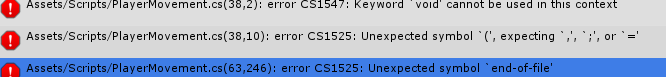# Can I make it work like this.

Looks weird (I think) Is there anyway to make the Jump from the wall to have a different force/power?``````using System.Collections;
using System.Collections.Generic;
using UnityEngine;

public class PlayerMovement : MonoBehaviour
{

public int playerspeed = 10;
public int playerJumpPower = 1250;
public float moveX;
public bool isGrounded;
public bool isonWall;

void Update()

{
PlayerMove ();
}

void PlayerMove()
{
//Controls
moveX = Input.GetAxis ("Horizontal");
if (Input.GetButtonDown ("Jump") && isGrounded == true) {
Jump ();
} else if (Input.GetButtonDown ("Jump") && isonWall == true) {
Jump ();
}

//Physics

gameObject.GetComponent<Rigidbody2D> ().velocity = new Vector2 (moveX * playerspeed, gameObject.GetComponent<Rigidbody2D> ().velocity.y);

void Jump()
{
//Jumping Code
isGrounded = false;

}

void OnCollisionEnter2D(Collision2D col)
{
if (col.gameObject.tag == "ground")
{
isGrounded = true;
}

else if (col.gameObject.tag == "Wall")
{
isonWall = true;
}

}
``````

Sure, just use call another method when you find a wall jump. Oh and you were missing some brackets, that’s why you had all those errors by the way.

``````using System.Collections;
using System.Collections.Generic;
using UnityEngine;

public class PlayerMovement : MonoBehaviour
{

public int playerspeed = 10;
public int playerJumpPower = 1250;
public int playerJumpFromWallPower = 1000;
public float moveX;
public bool isGrounded;
public bool isonWall;

void Update()

{
PlayerMove();
}

void PlayerMove()
{
//Controls
moveX = Input.GetAxis("Horizontal");
if (Input.GetButtonDown("Jump") && isGrounded == true)
{
Jump();
}
else if (Input.GetButtonDown("Jump") && isonWall == true)
{
JumpFromWall();
}

//Physics

gameObject.GetComponent<Rigidbody2D>().velocity = new Vector2(moveX * playerspeed, gameObject.GetComponent<Rigidbody2D>().velocity.y);

}

void Jump()
{
//Jumping Code
isGrounded = false;

}

void JumpFromWall(){
isGrounded = false;
isonWall = false;
}

void OnCollisionEnter2D(Collision2D col)
{
if (col.gameObject.tag == "ground")
{
isGrounded = true;
}

else if (col.gameObject.tag == "Wall")
{
isonWall = true;
}

}
}
``````